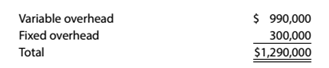Chapter 13, Problem 13.28E

Chapter
Section
Textbook Problem

Factory overhead cost variances Osceola Textiles Corporation began May with a budget for 45.000 hours of production in the Weaving Department. The department has a full capacity of 60,000 hours under normal business conditions. The budgeted overhead at the planned volumes at the beginning of May was as follows:The actual factory overhead was $1,428,000 for May. The actual fixed factor)' overhead was as budgeted. During May, the Weaving Department had standard hours at actual production volume of 52.000 hours.a.Determine the variable factory overhead controllable variance. b. Determine the fixed factory overhead volume variance. To determine (a) Concept Introduction: The variable factory overhead controlled variance is difference between the budgeted and actual variable factory overhead. The fixed factory overhead volume variance is the difference between the standard fixed factory overhead at actual production and at 100% of normal capacity. The sum of variable overhead controlled variance and fixed factory overhead volume variance is the factory overhead cost variance. The variable factory overhead controlled variance. Explanation In the given case following data is given: Actual factory overhead cost =$1,428,000

Budgeted variable factory overhead cost =$990,000 Budgeted fixed factory overhead cost =$300,000

Standard hours for 100% of normal capacity =60,000 hours

Standard hours for actual production =52,000 hours

Budgeted hours =45,000 hours

Budgeted variable factory overhead at 100% of normal capacity is:

Overhead=Budgeted variable factory overhead costBudgeted hours×Normal productive capacity=$990,00045,000×60,000=$1,320,000

Now, the calculation of variable overhead rate is as follows:

Variable factory overhead rate = Budgeted variable factory overhead cost at normal capacityNormal productive hours=\$1,

To determine

(b)

Concept Introduction:

The variable factory overhead controlled variance is difference between the budgeted and actual variable factory overhead.

The fixed factory overhead volume variance is the difference between the standard fixed factory overhead at actual production and at 100% of normal capacity.

The variable and fixed factory overhead controlled variance is that variance which does not change with change in volume.

The fixed factory overhead volume variance.

Still sussing out bartleby?

Check out a sample textbook solution.

See a sample solution

The Solution to Your Study Problems

Bartleby provides explanations to thousands of textbook problems written by our experts, many with advanced degrees!

Get Started

EXTERNAL EQUITY FINANCING Northern Pacific Heating and Cooling Inc. has a 6-month backlog of orders for its pat...

Fundamentals of Financial Management, Concise Edition (with Thomson ONE - Business School Edition, 1 term (6 months) Printed Access Card) (MindTap Course List)

(Oligopoly Power) What are three sources of oligopolies?

ECON: MICRO4 (New, Engaging Titles from 4LTR Press)

Why might purchasing power parity fail to hold?

Fundamentals of Financial Management (MindTap Course List)